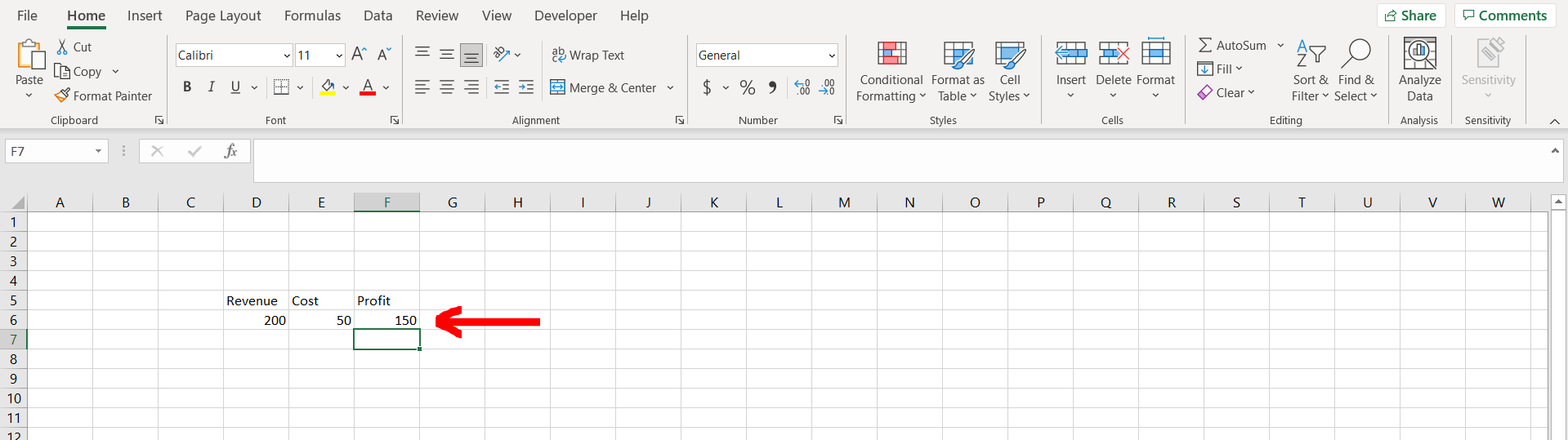# How to calculate profit in ExcelExcel offers a very interesting way to calculate profit. We can cater to this problem statement by subtracting cost from revenue. We can perform the below mentioned way to calculate profit in excel:

### Step 1 – Excel workbook with revenue and cost– Open the desired Excel workbook which contains revenue and cost

### Step 2 – Formula applied– Now subtract the cost from revenue as shown in the image above

### Step 3 – Profit calculated– We can see that the profit has been calculated.